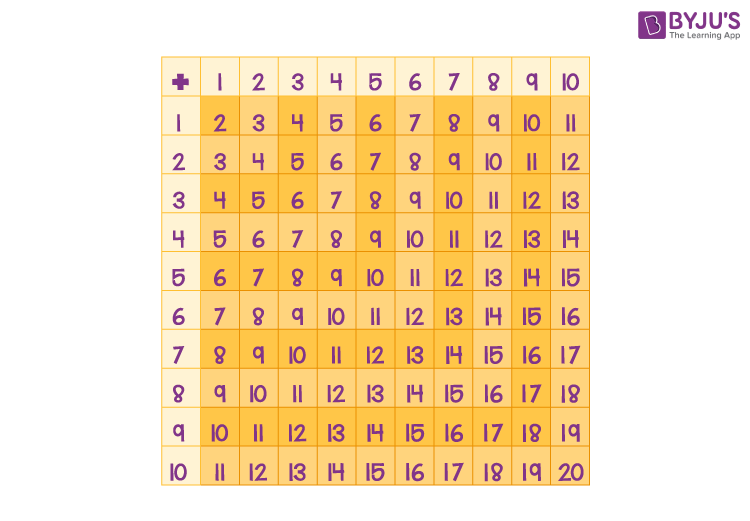Defend yourself better by mastering the science of immunity and vaccines. Secure your free spot, now! Defend yourself better by mastering the science of immunity and vaccines. Secure your free spot, now!

The term “Arithmetic addition” is used to describe adding two or more numbers together to form the addition table and the math symbols used to denote an addition operation is a plus sign. That is “ + “. For example 4 + 4.

The word “sum” also defines the same addition operation and we can use the summation symbol “Σ” for the series of numbers for addition.

The addition table chart for the numbers 1 to 10 can be represented as shown in the below figure.Here, the addition of two numbers can be identified by moving along the particular row and column, Suppose, the addition of 4 and 7 can be identified by moving along the row “4” and column “7”, where the intersection of these two will be the result, i.e. 11.

However, we can write the addition tables individually for all the numbers from 1 to 10. These can be written as:

 Addition Table for 1 Addition Table for 2 Addition Table for 3 Addition Table for 4 1 + 1 = 2 1 + 2 = 3 1 + 3 = 4 1 + 4 = 5 2 + 1 = 3 2 + 2 = 4 2 + 3 = 5 2 + 4 = 6 3 + 1 = 4 3 + 2 = 5 3 + 3 = 6 3 + 4 = 7 4 + 1 = 5 4 + 2 = 6 4 + 3 = 7 4 + 4 = 8 5 + 1 = 6 5 + 2 = 7 5 + 3 = 8 5 + 4 = 9 6 + 1 = 7 6 + 2 = 8 6 + 3 = 9 6 + 4 = 10 7 + 1 = 8 7 + 2 = 9 7 + 3 = 10 7 + 4 = 11 8 + 1 =9 8 + 2 = 10 8 + 3 = 11 8 + 4 =12 9 + 1 =10 9 + 2 = 11 9 + 3 = 12 9 + 4 =13 10 +1 = 11 10 + 2 = 12 10 + 3 = 13 10 + 4 = 14
 Addition Table for 5 Addition Table for 6 Addition Table for 7 Addition Table for 8 1 + 5 = 6 1 + 6 = 7 1 + 7 = 8 1 + 8 = 9 2 + 5 = 7 2 + 6 = 8 2 + 7 = 9 2 + 8 = 10 3 + 5 = 8 3 + 6 = 9 3 + 7 = 10 3 + 8 = 11 4 + 5 = 9 4 + 6 = 10 4 + 7 = 11 4 + 8 = 12 5 + 5 = 10 5 + 6 = 11 5 + 7 = 12 5 + 8 = 13 6 + 5 = 11 6 + 6 = 12 6 + 7 = 13 6 + 8 = 14 7 + 5 = 12 7 + 6 = 13 7 + 7 = 14 7 + 8 = 15 8 + 5 =13 8 + 6 =14 8 + 7 =15 8 + 8 =16 9 + 5 =14 9 + 6 =15 9 + 7 =16 9 + 8 =17 10 + 5 = 15 10 + 6 = 16 10 + 7 = 17 10 + 8 = 18
 Addition Table for 9 Addition table for 10 1 + 9 = 10 1 + 10 = 11 2 + 9 = 11 2 + 10 = 12 3 + 9 = 12 3 + 10 = 13 4 + 9 = 13 4 + 10 = 14 5 + 9 = 14 5 + 10 = 15 6 + 9 = 15 6 + 10 = 16 7 + 9 = 16 7 + 10 = 17 8 + 9 =17 8 + 10 = 18 9 + 9 =18 9 + 10 = 19 10 + 9 = 19 10 + 10 = 20

• Adding 0 to any number makes no difference.

For example : 4 + 0 = 4 ; 21 + 0 = 21

• The answer will be the same when you add a group of numbers together. It doesn’t matter about the order of sequence in the operation of addition.

Example : 1+2+3+4= 10 will be the same as the sequence 4+3+2+1=10

• To perform addition calculation for a longer list of numbers in an easy way such that arrange the numbers in a column and perform addition operation.
• 1 added to an even number that gives an added number
• Addition of two even numbers always results in an even number.
• 1 added to any number say, ‘x’, just gives you the next number after x.

Students can generate the addition table 1 to 10 for their learning and practice and it is used for all grade students. Here, you are provided with addition tables from 1 to 10 for reference. In the similar way, you can write it for for further tables.

For more related topics on addition, subtraction and multiplication tables, visit BYJU’S to learn in an easy and engaging way and watch interactive videos.